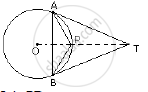Share

Ta and Tb Are Tangents to a Circle with Centre O from an External Point T. Ot Intersects the Circle at Point P. Prove that Ap Bisects the Angle Tab. - ICSE Class 10 - Mathematics

ConceptTangent Properties - If a Line Touches a Circle and from the Point of Contact, a Chord is Drawn, the Angles Between the Tangent and the Chord Are Respectively Equal to the Angles in the Corresponding Alternate Segments

Question

TA and TB are tangents to a circle with centre O from an external point T. OT intersects the circle at point P. Prove that AP bisects the angle TAB.

SolutionJoin PB.
In ΔTAP and ΔTBP,
TA = TB (tangents segments from an external points are equal in length)
Also, ∠ATP =  ∠BTP. (since OT is equally inclined with TA and TB) TP = TP (common)
⇒  ΔTAP ≅ ΔTBP (by SAS criterion of congruency)
⇒  ∠TAP =  ∠TBP (corresponding parts of congruent triangles are equal)
But ∠TBP =  ∠BAP (angles in alternate segments)
Therefore, ∠TAP = ∠BAP.
Hence, AP bisects  ∠TAB.

Is there an error in this question or solution?

Video TutorialsVIEW ALL 

Solution Ta and Tb Are Tangents to a Circle with Centre O from an External Point T. Ot Intersects the Circle at Point P. Prove that Ap Bisects the Angle Tab. Concept: Tangent Properties - If a Line Touches a Circle and from the Point of Contact, a Chord is Drawn, the Angles Between the Tangent and the Chord Are Respectively Equal to the Angles in the Corresponding Alternate Segments.
S